The goal is to generate 3D trees programatically rather than using a design tool. A tree is a complex model, containing thousand of vertices, which are both hard to create and inneficient to store. By saving just the information necessary to generate them we save memory and make their creation easier. The steps to accomplish this are:

• Create a language that allows specifying an L-System, and write a lexer and a parser for it.
• Interpret the language and create a 3D model from it.
• Give the model life: create branches and leaves.

The grammar of the L-System language is the following:

```LSystem ::= ( Rule )*

Rule ::= RuleName ParenthesizedNumber? ':' Actions ';'

Actions ::=
(
( f | '+' | '-' | '<' | '>' | 'v' | '^' ) ParenthesizedNumber?
|
( s | h | u | l | r ) ParenthesizedNumber
|
RuleName
|
Branch
)*

ParenthesizedNumber ::= '(' Number ')'

Branch ::= '[' Actions ']'

RuleName ::= [A-Z]

Number ::= [0-9]+ ( '.' [0-9]+ )?
```

A tree will be drawn by a turtle. The turtle starts at a certain position, for example (0, 0). The turtle follows the actions given by a rule of the L-System.

If it encounters f, it advances a step. +, -, <, >, v and ^ tell the turtle to turn clockwise or counterclockwise aroung a given axis relative to the turtle, for example up, left or head. A number between parenthesis indicates the ammount to step or turn. For example, f(2) advances two steps instead of one.

s, h, u, l and r are multipliers. s multiplies the current step by the given number. h, u and l multiplies the turn angle along each of the axis. r multiplies the trunk radius.

A branch tells the turtle to push it's state into a stack, do the actions inside the branch, and then pop the state. This effectively allows making many branches from a given point.

If the name of a rule is found, it is consumed (interpreted again by the turtle). Since this may lead to infinite recursion, a number of iterations is defined: each time a rule is consumed, the number of iterations is decremented, until no further rules are consumed.

To see this in action, I first wanted to know if it worked in a 2D environment: requestor pattern in action! A TurtleCommander interprets the rules, starting by an axiom (the name of the first rule to intepret). As it interprets the rules and actions, it notifies an ITurtle what to do. An ITurtle is an interface that has methods like Advance, RotateAlongAxis, MultiplyCurrentStep, etc. In this way, the intepretation of the rules is decoupled from their processing. A 2D ITurtle will ignore the rotation axis because there is only one in a 2D plane. Also, I started without trunk radius, so these calls are ignored.

Another important aspect is to give realism to the generated trees. If the rules are obeyed without modification, the trees will look too symmetric, too perfect. To fix this, we introduce randomness when intepreting an action, so a step or a rotation becomes a little longer or shorter. This is all handled by the ITurtle implemantation.

Here are some screenshots of 2D trees, together with their L-System, step, rotation angle and number of iterations:2D tree, 26 degrees A: f[+A][-A]; 1 iteration2D tree, 26 degrees A: f[+A][-A]; 2 iterations2D tree, 26 degrees A: f[+A][-A]; 3 iterations2D tree, 26 degrees A: f[+A][-A]; 4 iterations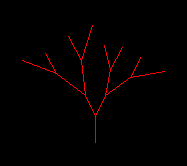2D tree, 26 degrees A: f[+A][-A]; 4 iterations with randomness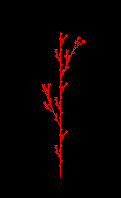A regular weed A: fA [+fA] fA [-fA] fA; 3 iterationsA simple bush A: fA fA +[+fA -fA -fA] - [-fA +fA +fA]; 3 iterations

The next step is to make it 3D. I made another implementation of ITurtle which now understood every action except the radius change. It creates 3D instead of 2D lines. Here's a screenshot of some early trees: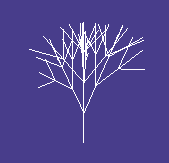A: f[+A][-A][A]; A: f[+A][-A][A]; 4 iterations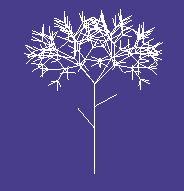A more realistic tree A: f [-s(0.6)C] s(0.5) f [+^C] f s(2) [+B][-B][^B][vB]; B: s(0.7)f[+B][-B][^B][vB]; C: f; 5 iterations

Next, to draw textured cylinders instead of lines: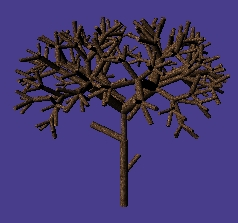3D tree with textures trunks A: f [-s(0.6)C] s(0.5) f [+^C] f s(2) [+B][-B][^B][vB]; B: s(0.7)f[+B][-B][^B][vB]; C: f; 5 iterations

Finally, it starts looking like a real tree. This is being drawn with the DirectX API, but without using vertex shaders and pixel shaders.

The next step is to make use of vertex and pixel shaders to give branches a more realistic appearance. To do this, instead of just sticking branches together, I stick them and twist them. Also, radius changes are now interpreted. The result is this: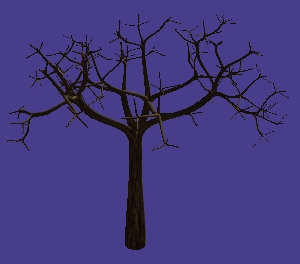3D tree, almost real A: f r(0.6) [+B][-B][^B][vB]; B: s(0.6) f r(0.5) [+B][-B][^B][vB]; 5 iterations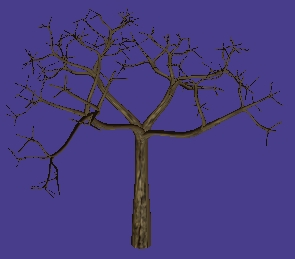3D tree, almost real (2)

Nice, huh? Finally, I use a billboard technique to draw leaves in the last branches. Here are some screenshots of trees: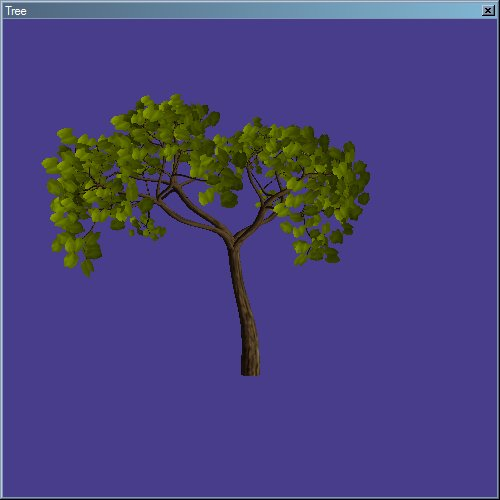3D tree 1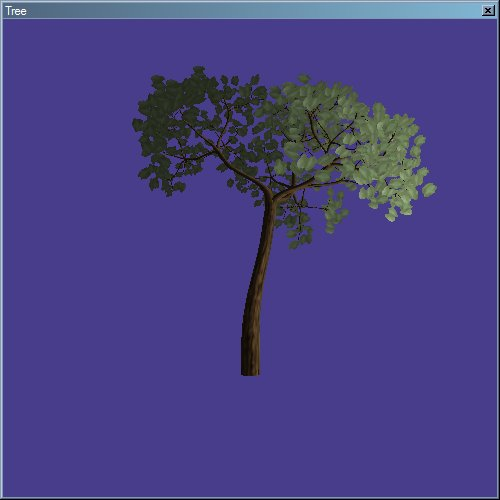3D tree 2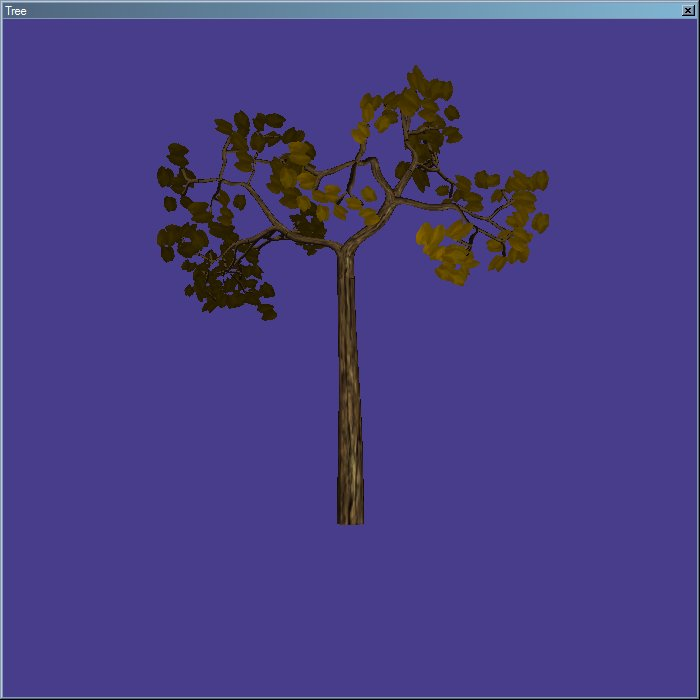3D tree 3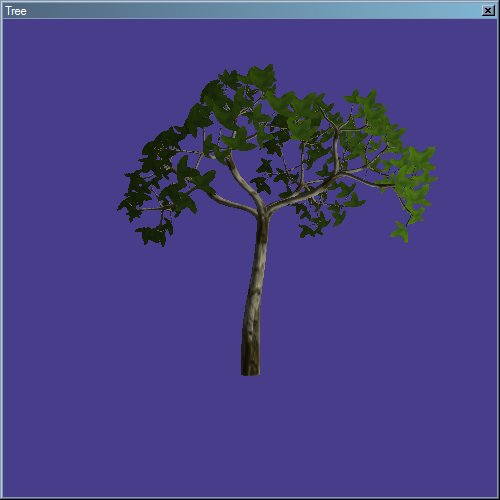3D tree 4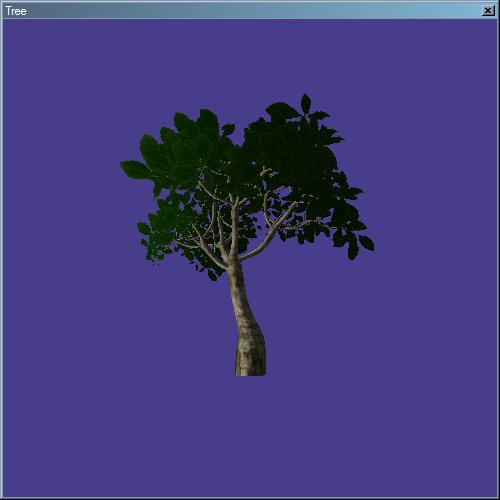3D tree 5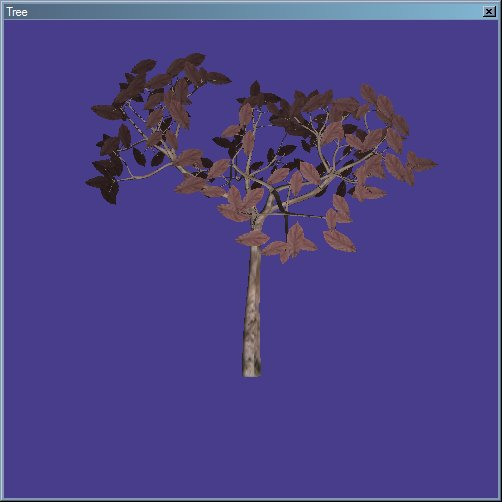3D tree 6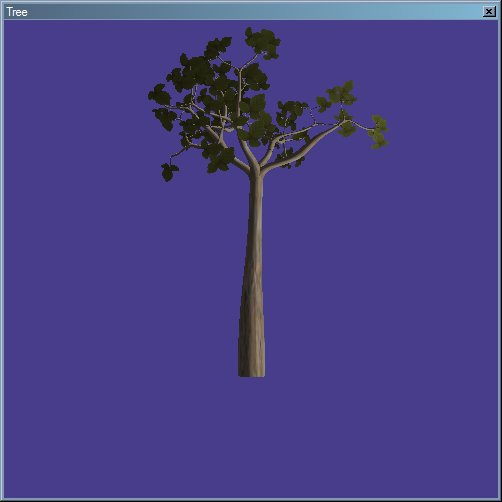3D tree 7

I really enjoyed how the look of the trees changed as I added, little by little, more details to them.